• 数据结构员工信息管理系统，课程设计用，用MFC和C++实现
• 6/10/2020 成 绩 成 绩 信计 一 12信计2013-2014 一 12 2013-2014 数 据 结 构 课 程 设 计 数 据 结 构 课 程 设 计
• 数据结构课程设计，一个简单的基于二叉排序树的员工信息管理系统
• 员工管理数据结 构员工管理系统 壹设计题目 [问题描述] 每个员工信息包括编号姓名性别出生年月学历职务 电话住址等系统能够完成员工信息的查询更新插 入删除排序等功能 [基本要求] 1 排序按不同关键字对所有员工的...
• 这是用C语言编写的企业员工信息管理系统，本系统是我们《数据结构》课程的课程设计题目
• 浙江工商大学计算机与信息工程学院 数据结构实验大作业报告 专 业 电 子 商 务 班 级 商 务1202 学 号 1212200206 姓 名 陈志伟 指导教师 韩建伟 2013 年 5 月 一问题分析 职工管理系统是一个工作单位不可缺少的管理...
• 数据结构”课程设计报告 职工信息管理系统 课程设计任务与要求： [问题描述] 每个员工的信息包括：编号、姓名、性别、出生年月、学历、职务、电话、住址等。系统能够完成员工信息的查询、更新、插入、删除、排序等...


“数据结构”课程设计报告

系 （院）：           信息工程学院
设计题目：           员工管理系统
专业班级：          计算机科学与技术1401B
小组成员：     杜康辉 1434110115
小组成员：     吴孝乐 1434110147
小组成员：     张广然 1434110133
指导教师：              汪洋
完成时间：       2015~2016学年第一学期

目录
第一部分 课程设计目的 1
第二部分 课程设计任务与要求 1
一、问题描述 1
二、基本要求 1
三、测试数据 1
四、实现提示 1
第三部分 程序设计流程  2
一、需求分析 2
二、概要设计 2
三、详细设计 3
四、设计与调试分析 9
五、用户手册 9
六、测试成果 10
七、附录(原程序清单) 14
八、课程设计心得 30
第四部分 参考文献 31

² 课程设计目的  1、能够灵活地应用所学数据结构知识，根据加工数据对象的特征，选择适当的数据结构、存贮结构及相应算法，独立完成问题分析，结合数据结构理论知识，编写程序求解指定问题。 2. 初步掌握各种算法在时间和空间的分析技巧；初步掌握软件开发过程的问题分析、系统设计、程序编码、测试等基本方法和技能； 3. 能够进行算法设计和程序设计，并且使所设计的程序结构清楚，正确易读，并上机调试通过；提高综合运用所学的理论知识和方法独立分析和解决问题的能力。 4.用系统的观点和软件开发一般规范进行软件开发，巩固、深化学生的理论知识，提高编程水平，并在此过程中培养他们严谨的科学态度和良好的工作作风。 5. 培养较强的实习和实践能力；较强的分析问题和解决问题的能力。  ² 课程设计任务与要求： [问题描述] 每个员工的信息包括：编号、姓名、性别、出生年月、学历、职务、电话、住址等。系统能够完成员工信息的查询、更新、插入、删除、排序等功能。 [基本要求] （1） 排序：按不同关键字，对所有员工的信息进行排序。 （2） 查询：按特定条件查找员工。 （3） 更新：按编号对某个员工的某项信息进行修改。 （4） 插入：加入新员工的信息。 （5） 删除：按编号删除已离职的员工的信息。   [测试数据]   每个员工的信息：编号、姓名、性别、出生年月、学历、职务、电话、住址等。 [实现提示] 通过相关操作会使输入显示在屏幕上。 一 需求分析 1.设计“职工信息管理系统”，使其实现对职工信息的建立、查询、删除、修改和遍历操作。 2.数据包括：职工号、姓名、性别、年龄、学历、工资、住址、电话等。 3.能够对其中的职工信息进行修改和删除。 4.能够实现对所有职工信息的遍历。 5.能够实现查找功能： ①完成按职工的职工号查询职工的相关信息，并显示。 ②完成按职工的学历查询职工的相关信息，并显示。     ③完成按职工的电话号码查询职工的相关信息，并显示。   二 概要设计 1.用结构体指针数组来实现。 2.实现对职工基本信息的录入。 3.实现对职工基本信息的浏览。 4.实现对职工基本信息的查找。 5.实现对职工基本信息的删除。 6.实现对职工基本信息的添加。 7.实现对职工基本信息的修改。 系统功能模块结构图        /*定义存储单元基本信息的结构*/ struct employee           //定义结构体employee {  int num;                 char name;          //职工姓名 char sex;               //职工性别 char xueli;          //学历 int wage;              //年龄 char addr;         //住址 long int tel;            //电话 }em;  三 详细设计           四 设计与调试分析 调试过程中会遇到输入超出范围等容易因为手误犯的错误，这一点一定要注意，编写程序之前，首先要对如何设计程序有一个清晰的思路,清楚怎么去设计,然后系统分析与系统设计，最后是代码设计与调试。这些都是编写课程设计的步骤，同时，还得注意要把程序编写得易懂，让界面清楚。 设计过程中，我们根据以往学过的数据结构的概念、理论和方法，设计程序的基本步骤，来完成这个应用，同时也加深了我们对数据结构的掌握程度。 经过本次设计，我们的程序设计能力得到了很大的提高，但相对于那些高手来说，还有很大差距，仍需继续努力。而且，我还懂得要增强团队合作，要有合作精神，这样才会有意想不到的结果。   五 用户手册 提示操作：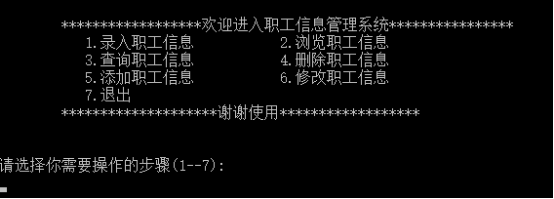分析：提示开始输入数据的操作！ 1.录入职工信息操作：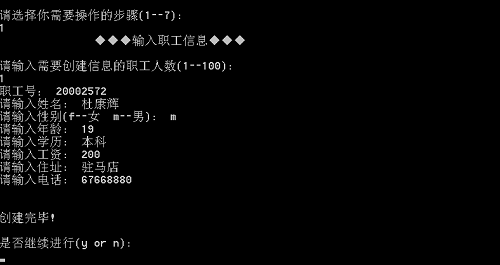分析：录入职工信息的数据！ 2.浏览职工信息操作：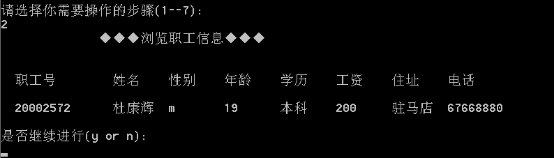分析：浏览职工信息 3.查询职工信息操作：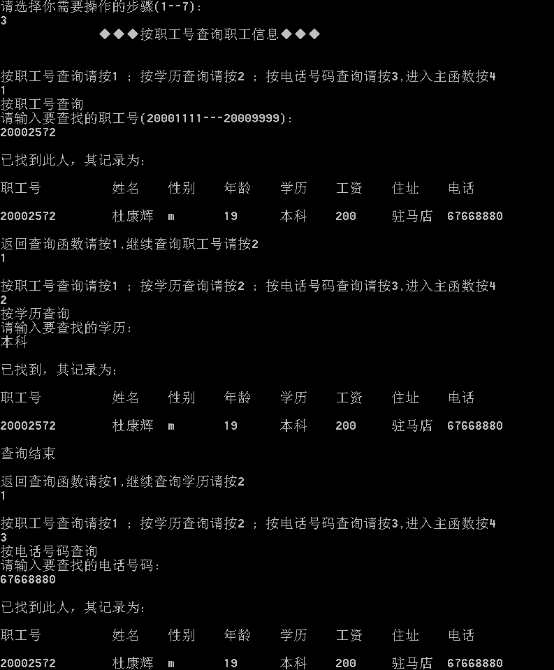分析：输入要查询的职工号，学历，电话号码！ 4.删除职工信息操作：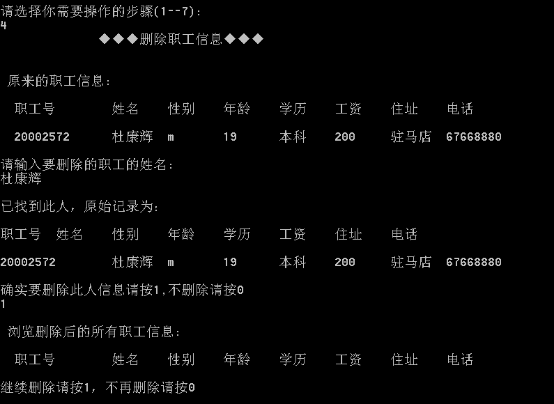分析：输入要删除的职工姓名！ 5.添加职工信息操作：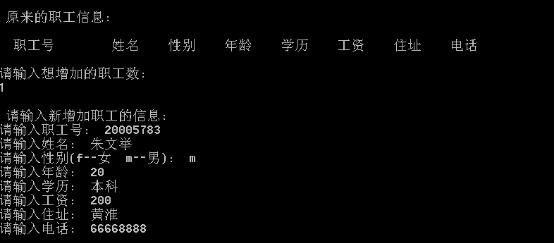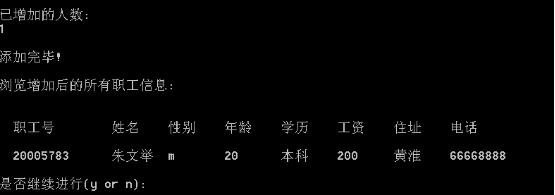分析：输入要添加的职工信息！ 6.修改职工信息操作：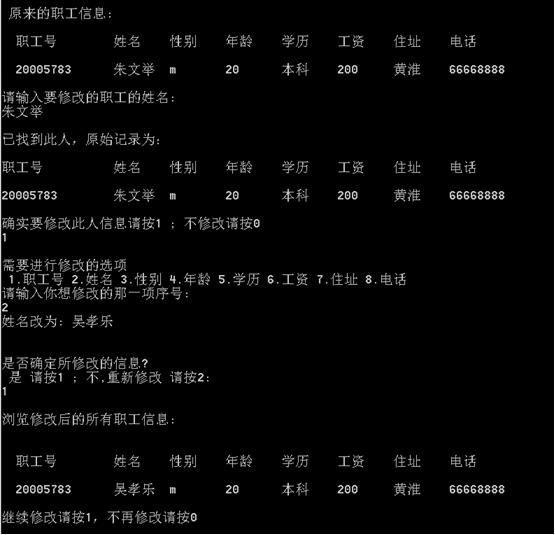分析：输入要修改的职工的姓名！   注：任意输入一个数据后进入系统，然后根据系统提示操作即可。 六 测试成果   七 附录（源程序清单） //****************************************************** //*  程 序 名: 职工信息管理系统                                   //*  作    者: 杜康辉、吴孝乐、张广然 //*  编制时间: 2016年1月2日                                   //*  主要功能: 职工信息管理                                   //****************************************************** #include <stdio.h> #include <windows.h> #include <time.h> #include <string.h> #define N 100 struct employee {     int num; char name; char sex;   int age; char xueli;     int wage; char addr; long int tel; }em;        /*定义一个结构体*/ void menu(); void input(); void save(int); void display(); void del(); void add(); void search(); void search_num(); void search_xueli(); void search_tel(); void modify();  /*定义各函数*/   void menu()   /*菜单函数*/ { //printf("              ☆☆☆计算机科学与技术☆☆☆\n");     //printf("\n");     //printf("                                   ∮1401B班 ∮\n");     printf("\n"); printf("        ******************欢迎进入职工信息管理系统****************\n"); printf("           1.录入职工信息"); printf("           2.浏览职工信息\n"); printf("           3.查询职工信息"); printf("           4.删除职工信息\n");     printf("           5.添加职工信息"); printf("           6.修改职工信息\n"); printf("           7.退出\n"); printf("        ********************谢谢使用******************\n");     printf("\n");     printf("\n");  } int main() {    menu();  /*调用菜单函数*/ int n,flag; char a; do { printf("请选择你需要操作的步骤(1--7):\n"); scanf("%d",&n); if(n>=1&&n<=7) { flag=1; break; } else { flag=0; printf("您输入有误，请重新选择!"); } }     while(flag==0);     while(flag==1) { switch(n) { case 1:printf("               ◆◆◆输入职工信息◆◆◆\n");printf("\n");input();break; case 2:printf("              ◆◆◆浏览职工信息◆◆◆\n");printf("\n");display();break; case 3:printf("              ◆◆◆按职工号查询职工信息◆◆◆\n");printf("\n");search();break;  case 4:printf("              ◆◆◆删除职工信息◆◆◆\n");printf("\n");del();break; case 5:printf("              ◆◆◆添加职工信息◆◆◆\n");printf("\n");add();break; case 6:printf("               ◆◆◆修改职工信息◆◆◆\n");printf("\n");modify();break; case 7:exit(0);break; default :break; } getchar(); printf("\n"); printf("是否继续进行(y or n):\n"); scanf("%c",&a); if(a=='y') { flag=1; system("cls");  /*清屏*/ menu(); /*调用菜单函数*/ printf("请再次选择你需要操作的步骤(1--6):\n"); scanf("%d",&n); printf("\n"); }  else  exit(0); } }   void input()   /*录入函数*/ { int i,m; printf("请输入需要创建信息的职工人数(1--100):\n"); scanf("%d",&m); for (i=0;i<m;i++) { printf("职工号： "); srand((int)time(0)); em[i].num=rand()%10000+20000000; if(em[i].num!=em[i-1].num) printf("%8d ",em[i].num); printf("\n"); printf("请输入姓名:  "); scanf("%s",em[i].name); getchar(); printf("请输入性别(f--女  m--男):  "); scanf("%c",&em[i].sex); printf("请输入年龄:  "); scanf("%d",&em[i].age); printf("请输入学历:  "); scanf("%s",em[i].xueli); printf("请输入工资:  "); scanf("%d",&em[i].wage); printf("请输入住址:  "); scanf("%s",em[i].addr);         printf("请输入电话:  "); scanf("%d",&em[i].tel); printf("\n");  } printf("\n创建完毕!\n"); save(m); } void save(int m)  /*保存文件函数*/ { int i; FILE*fp; if ((fp=fopen("employee.txt","w"))==NULL)  /*创建文件并判断是否能打开*/ { printf ("cannot open file\n"); exit(0); } for (i=0;i<m;i++) /*将内存中职工的信息输出到磁盘文件中去*/ if (fwrite(&em[i],sizeof(struct employee),1,fp)!=1) printf("file write error\n"); fclose(fp); }   int load()  /*导入函数*/ { FILE*fp; int i=0; if((fp=fopen("employee.txt","r"))==NULL) { printf ("cannot open file\n"); exit(0); } else  { do  { fread(&em[i],sizeof(struct employee),1,fp); i++; } while(feof(fp)==0); } fclose(fp); return(i-1); } void display()  /*浏览函数*/ { int i; int m=load(); printf("\n  职工号\t姓名\t性别\t年龄\t学历\t工资\t住址\t电话   \n"); for(i=0;i<m;i++) /*m为输入部分的职工人数*/ printf("\n  %d\t%s\t%c\t%d\t%s\t%d\t%s\t%ld\n",em[i].num,em[i].name,em[i].sex,em[i].age,em[i].xueli,em[i].wage,em[i].addr,em[i].tel);   } void del()   /*删除函数*/ {     int m=load(); int i,j,n,t,flag; char name; printf("\n 原来的职工信息:\n");     display();   /* 调用浏览函数*/     printf("\n"); printf("请输入要删除的职工的姓名:\n"); scanf("%s",name); for(flag=1,i=0;flag&&i<m;i++) { if(strcmp(em[i].name,name)==0) { printf("\n已找到此人，原始记录为：\n");             printf("\n职工号\t姓名\t性别\t年龄\t学历\t工资\t住址\t电话   \n"); printf("\n%d\t%s\t%c\t%d\t%s\t%d\t%s\t%ld\n",em[i].num,em[i].name,em[i].sex,em[i].age,em[i].xueli,em[i].wage,em[i].addr,em[i].tel);               printf("\n确实要删除此人信息请按1,不删除请按0\n"); scanf("%d",&n);             if(n==1)   /*如果删除，则其他的信息都往上移一行*/ { for(j=i;j<m-1;j++) { strcpy(em[j].name,em[j+1].name); em[j].num=em[j+1].num; em[j].sex=em[j+1].sex; em[j].age=em[j+1].age; strcpy(em[j].xueli,em[j+1].xueli); em[j].wage=em[j+1].wage;   strcpy(em[j].addr,em[j+1].addr); em[j].tel=em[j+1].tel; } flag=0; } } } if(!flag) m=m-1; else printf("\n对不起，查无此人!\n");     printf("\n 浏览删除后的所有职工信息:\n");     save(m);     /*调用保存函数*/     display();  /*调用浏览函数*/ printf("\n继续删除请按1，不再删除请按0\n"); scanf("%d",&t); switch(t) {     case 1:del();break;     case 0:break;     default :break; } } void add()/*添加函数*/ { FILE*fp; int n;     int count=0;     int i;     int m=load();     printf("\n 原来的职工信息:\n");     display();   /* 调用浏览函数*/     printf("\n");     fp=fopen("employee.txt","a");     printf("请输入想增加的职工数:\n"); scanf("%d",&n);     for (i=m;i<(m+n);i++) { printf("\n 请输入新增加职工的信息:\n"); printf("请输入职工号:  "); srand((int)time(0)); em[i].num=rand()%10000+20000000; if(em[i].num!=em[i-1].num) printf("%8d ",em[i].num); printf("\n");         printf("请输入姓名:  "); scanf("%s",em[i].name);         getchar();         printf("请输入性别(f--女  m--男):  "); scanf("%c",&em[i].sex);         printf("请输入年龄:  "); scanf("%d",&em[i].age); printf("请输入学历:  "); scanf("%s",em[i].xueli); printf("请输入工资:  "); scanf("%d",&em[i].wage); printf("请输入住址:  "); scanf("%s",em[i].addr);         printf("请输入电话:  "); scanf("%d",&em[i].tel);         printf("\n"); count=count+1; printf("已增加的人数:\n"); printf("%d\n",count); }     printf("\n添加完毕!\n");      m=m+count; printf("\n浏览增加后的所有职工信息:\n"); printf("\n"); save(m);     display(); fclose(fp); } void search()/*查询函数*/ {     int t,flag; do { printf("\n按职工号查询请按1 ; 按学历查询请按2 ; 按电话号码查询请按3,进入主函数按4\n"); scanf("%d",&t); if(t>=1&&t<=4) { flag=1; break; } else { flag=0; printf("您输入有误，请重新选择!"); } }     while(flag==0);     while(flag==1) { switch(t) {  case 1:printf("按职工号查询\n");search_num();break; case 2:printf("按学历查询\n");search_xueli();break; case 3:printf("按电话号码查询\n");search_tel();break; case 4:main();break; default:break; } } } void search_num() { int num; int i,t; int m=load(); printf("请输入要查找的职工号(20001111---20009999):\n"); scanf("%d",&num); for(i=0;i<m;i++) if(num==em[i].num) {   printf("\n已找到此人，其记录为：\n"); printf("\n职工号\t\t姓名\t性别\t年龄\t学历\t工资\t住址\t电话   \n"); printf("\n%d\t%s\t%c\t%d\t%s\t%d\t%s\t%ld\n",em[i].num,em[i].name,em[i].sex,em[i].age,em[i].xueli,em[i].wage,em[i].addr,em[i].tel);   break; } if(i==m)   printf("\n查询结束\n"); printf("\n"); printf("返回查询函数请按1,继续查询职工号请按2\n"); scanf("%d",&t); switch(t) {  case 1:search();break; case 2: break; default:break; } } void search_xueli() { char xueli; int i,t; int m=load(); printf("请输入要查找的学历:\n"); scanf("%s",xueli); for(i=0;i<m;i++) if(strcmp(em[i].xueli,xueli)==0) { printf("\n已找到，其记录为：\n"); printf("\n职工号\t\t姓名\t性别\t年龄\t学历\t工资\t住址\t电话   \n"); printf("\n%d\t%s\t%c\t%d\t%s\t%d\t%s\t%ld\n",em[i].num,em[i].name,em[i].sex,em[i].age,em[i].xueli,em[i].wage,em[i].addr,em[i].tel);   } if(i==m)  printf("\n查询结束\n"); printf("\n"); printf("返回查询函数请按1,继续查询学历请按2\n"); scanf("%d",&t); switch(t) {  case 1:search();break; case 2:break; default :break; } } void search_tel() { long int tel; int i, t; int m=load(); printf("请输入要查找的电话号码:\n"); scanf("%ld",&tel); for(i=0;i<m;i++) if(tel==em[i].tel) {   printf("\n已找到此人，其记录为：\n"); printf("\n职工号\t\t姓名\t性别\t年龄\t学历\t工资\t住址\t电话   \n"); printf("\n%d\t%s\t%c\t%d\t%s\t%d\t%s\t%ld\n",em[i].num,em[i].name,em[i].sex,em[i].age,em[i].xueli,em[i].wage,em[i].addr,em[i].tel);   break; } if(i==m)   printf("\n查询结束\n"); printf("\n"); printf("返回查询函数请按1,继续查询电话号码请按2\n"); scanf("%d",&t); switch(t) {  case 1:search();break; case 2:break; default :break; } } void modify() /*修改函数*/ {     int num; char name; char sex;   int age; char xueli;     int wage; char addr;     long int tel;     int b,c,i,n,t,flag;  int m=load(); /*导入文件内的信息*/     printf("\n 原来的职工信息:\n");     display();   /* 调用浏览函数*/ printf("\n");     printf("请输入要修改的职工的姓名:\n"); scanf("%s",name); for(flag=1,i=0;flag&&i<m;i++) { if(strcmp(em[i].name,name)==0) { printf("\n已找到此人，原始记录为：\n");             printf("\n职工号\t\t姓名\t性别\t年龄\t学历\t工资\t住址\t电话   \n"); printf("\n%d\t%s\t%c\t%d\t%s\t%d\t%s\t%ld\n",em[i].num,em[i].name,em[i].sex,em[i].age,em[i].xueli,em[i].wage,em[i].addr,em[i].tel);               printf("\n确实要修改此人信息请按1 ; 不修改请按0\n"); scanf("%d",&n);             if(n==1) { printf("\n需要进行修改的选项\n 1.职工号 2.姓名 3.性别 4.年龄 5.学历 6.工资 7.住址 8.电话\n");   printf("请输入你想修改的那一项序号:\n");   scanf("%d",&c);   if(c>8||c<1)   printf("\n选择错误，请重新选择!\n");   } flag=0; }  }     if(flag==1) printf("\n查询结束\n");       do   { switch(c)      /*因为当找到第i个职工时,for语句后i自加了1,所以下面的应该把改后的信息赋值给第i-1个人*/ {   case 1:printf("职工号改为: "); scanf("%d",&num); em[i-1].num=num; break; case 2:printf("姓名改为: "); scanf("%s",name); strcpy(em[i-1].name,name); break;  case 3:printf("性别改为: "); getchar();scanf("%c",&sex);     em[i-1].sex=sex; break; case 4:printf("年龄改为: "); scanf("%d",&age); em[i-1].age=age; break; case 5:printf("学历改为: "); scanf("%s",xueli); strcpy(em[i-1].xueli,xueli); break;    case 6:printf("工资改为: "); scanf("%d",wage); break;  case 7:printf("住址改为: "); scanf("%s",addr); strcpy(em[i-1].addr,addr); break;   case 8:printf("电话改为: "); scanf("%ld",&tel); em[i-1].tel=tel; break;   }          printf("\n"); printf("\n是否确定所修改的信息?\n 是 请按1 ; 不,重新修改 请按2:  \n");          scanf("%d",&b); }   while(b==2); printf("\n浏览修改后的所有职工信息:\n"); printf("\n"); save(m); display(); printf("\n继续修改请按1，不再修改请按0\n"); scanf("%d",&t); switch(t) {     case 1:modify();break;     case 0:break;     default :break; } }   八.课程设计心得     通过这次课程设计，增加了我们对软件技术的了解，虽然还不明确软件技术包含的具体内容，但从学习数据结构这门课程开始，已发现程序设计的好处，它对我们数学的学习也有很大的帮助。在学习数据结构的过程中也学到和巩固了许多计算机应用基础知识，对计算机的机体也有了一个较为详细的了解。在具体操作中对这学期所学的数据结构理论知识得到巩固加强，达到实训的目的，也发现自己的不足之处，为我们以后的数据结构学习奠定了基础，同时体会到数据结构具有的语句简洁，使用灵活，执行效率高等特点。发现上机操作的重要作用，特别是对数组和循环有了深刻的理解。通过实际操作，学会 数据结构程序编程的基本步骤、基本方法，开发了自己的逻辑思维能力，培养了分析问题、解决问题的能力。 由于本课题中的许多知识点都没有学过都要靠自己到课外的资料中去查找。在用的时候难免出现这样那样的错误。 这几天，在指导教师汪洋的精心教导和队友们的齐力合作下，我们终于完成了这次课程设计。通过此次课程设计，我们学会了如何用数据结构的知识编写一个简单的应用程序，受益匪浅。 这次编程后，我也体会到编程是不容易的。以前还以为老师是开玩笑的，没想到竟然是真的，但是，看到自己编写出来的程序，心里也是快乐的。和队友在编写过程中，大家相互合作，为把程序写好而共同努力着。程序编写完成后，我们并没有得到意想的结果，而是一遍又一遍地调试和完善它，绞尽脑汁，终于，我们完成了。 做任何事都是不容易的，只要我们尽力去做，努力就行！天道酬勤！加油！ 以后要再接再厉，编出更多的、更好的、更实用的程序，所以从现在起要更加努力地学习！ 参考文献 数据结构 耿国华 著 高等教育出版社 程序设计基础（第三版） 吴文虎 徐明星 著 清华大学出版社


展开全文c语言 设计 c cpp
• 数据结构课程设计之学生宿舍信息管理系统，宿舍对于大学生在校生活来说相当于家的存在，而宿舍管理又是学校后勤管理的重要环节，如何直观的了解宿舍的入住情况和每位同学的住宿位置是提高工作效率的重要课题，根据...
一、问题陈述
宿舍对于大学生在校生活来说相当于家的存在，而宿舍管理又是学校后勤管理的重要环节，如何直观的了解宿舍的入住情况和每位同学的住宿位置是提高工作效率的重要课题，根据我们所学的C语言和数据结构课程中有关链表的内容，为宿舍管理人员编写宿舍管理查询软件，就可以轻松满足实现上述需求。 任务：
为宿舍管理人员编写一个宿舍管理查询软件, 程序设计要求：
采用交互工作方式可按关键字（姓名、学号、房号）进行排序 查询菜单: (用二分查找实现以下操作)
按姓名查询按学号查询按房号查询 打印任一查询结果（可以连续操作）
二、概要设计
2.1 概要简述
根据系统要求，即本系统具有信息的录入，显示，排序显示、查找，插入、删除、结束程序等功能，先设计出详细的系统流程图，然后将源代码输入程序，进行编译调试即可。 程序总体分10个项目：输入记录、显示记录、按姓名排序并显示、按房间号排序并显示 、按学号排序并显示 、按姓名查找并显示 、按房间号查找并显示 、按学号查找并显示、插入一条记录按学号排序并显示以及结束程序。
2.2 线性表存储结构表示
typedef struct {
char name;
int num;            //学号和房号都为整型
int room;
} stu;

typedef struct {
int length; //当前长度
stu *elem;  //存储空间基址
int listsize;  //当前分配的存储容量

2.3 详细设计
2.3.1 系统流程图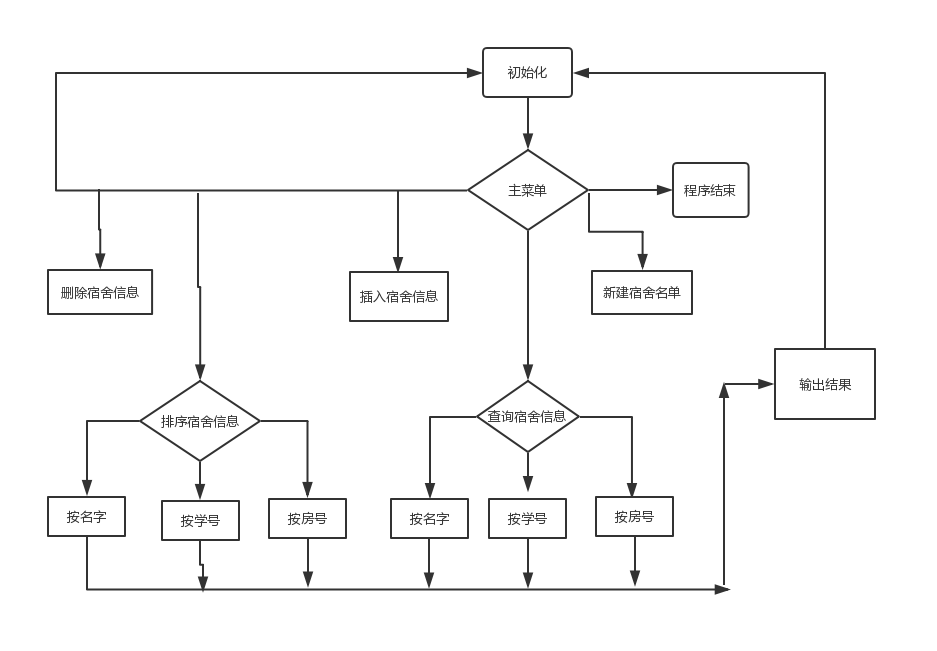2.3.2 三种排序方法及二分查找法
2.3.2.1 冒泡排序（按姓名排序）
//按姓名排序（采用冒泡排序）
int i, j;
stu temp;
for (i = 0; i<L.length - 1; i++)
for (j = 0; j<L.length-1-i; j++)
if (strcmp(L.elem[j].name, L.elem[j+1].name)>0) {
temp = L.elem[j];
L.elem[j] = L.elem[j+1];
L.elem[j+1] = temp;
}
}

2.3.2.2 折半插入排序（按学号排序）
//按学号排序（采用折半插入排序）
int i, j, mid, low, high;
stu temp;
for (i = 1; i < L.length; i++) {
if(L.elem[i].num<L.elem[i-1].num) {
temp = L.elem[i];
low = 0;
high = i-1;
while (low <= high) {
mid = (low + high) / 2;
if (temp.num < L.elem[mid].num)
high = mid - 1;
else
low = mid + 1;
}
for (j = i - 1; j >= high+1; j--)
L.elem[j+1]=L.elem[j];
L.elem[high+1]=temp;
}
}
}

2.3.2.3 简单选择排序（按房号排序）
//按房号排序（采用简单选择排序）
int i,j,k;
stu temp;
for(i=0; i<L.length-1; i++) {
k=i;
for(j=i+1; j<L.length; j++)
if(L.elem[j].room<L.elem[k].room)
k=j;
if(k!=i){
temp = L.elem[i];
L.elem[i] = L.elem[k];
L.elem[k] = temp;
}
}
}

2.3.2.4 二分查找法（以按姓名查找为例）
//按姓名从小到大查找（采用二分查找）
if (L.length == 0) {
printf("已无学生记录!\n");
Ret();
} else {
int low = 0, high = L.length, mid, flag = 0;
printf("\n");
printf("按姓名查找-->请输入要查找的姓名:");
char a, ch;
scanf("%s", a);
while (low <= high) {
mid = (low + high) / 2;
if (strcmp(a, L.elem[mid].name) == 0) {
flag = 1;
break;
} else if (strcmp(a, L.elem[mid].name)>0)
low = mid + 1;
else
high = mid - 1;
}
if (flag == 1) {
printf("查找成功-->该学生信息为:\n");
printf("姓名       学号    房号\n");
printf("%-10s %-2d %-5d\n", L.elem[mid].name, L.elem[mid].num, L.elem[mid].room);
if (Select())
search1(L);
else {
system("cls");
}
} else {
printf("该学生不存在!");
if (Select())    search1(L);
else {
system("cls");
}
}
}
}

三、测试与运行
3.1 系统界面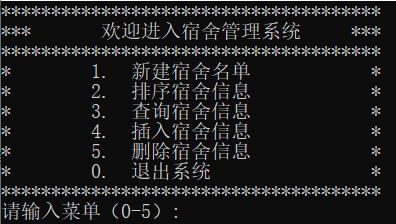3.2 新建宿舍名单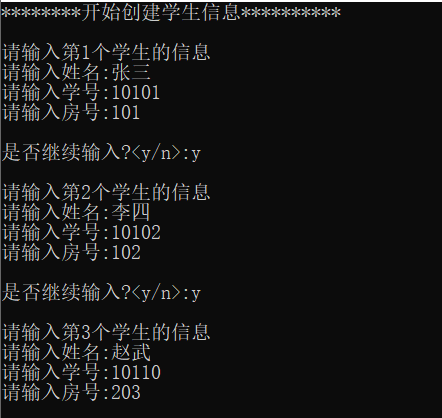3.3 排序（以姓名排序为例）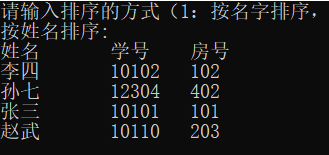3.4 查询（以学号查询为例）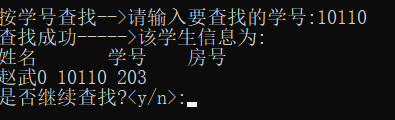3.5 插入学生信息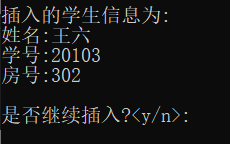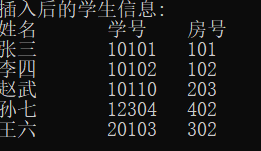3.6 删除学生信息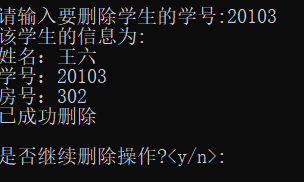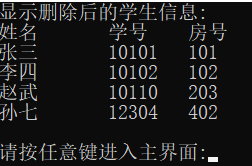四、代码实现
#include<stdio.h>
#include<stdlib.h>
#include<string.h>
#include<windows.h>
#define N 40 //线性表存储空间的初始分配量
#define increase 10 //线性表存储空间的分配量增量
int choice;  //定义全局变量
typedef struct {
char name;
int num;            //学号和房号都为整型
int room;
} stu;
stu stud;
typedef struct {
int length; //当前长度
stu *elem;  //存储空间基址
int listsize;  //当前分配的存储容量

//线性表初始化
L.length = 0;
L.elem = (stu *)malloc(N * sizeof(stu));
L.listsize = N;
}

//操作菜单
printf( "**************************************\n" );
printf( "***       欢迎进入宿舍管理系统     ***\n" );
printf( "**************************************\n" );
printf( "*        1.  新建宿舍名单            *\n" );
printf( "*        2.  排序宿舍信息            *\n" );
printf( "*        3.  查询宿舍信息            *\n" );
printf( "*        4.  插入宿舍信息            *\n" );
printf( "*        5.  删除宿舍信息            *\n" );
printf( "*        0.  退出系统                *\n" );
printf( "**************************************\n" );
printf("请输入菜单（0-5）:");
scanf("%d", &choice);
if (choice<0 || choice>5) {
system("cls");
printf("输入数字不对,请重新!\n");
printf("\n");
}

}

//打印学生信息
int i;
printf("姓名       学号    房号\n");
for (i = 0; i<L.length; i++)
printf("%-10s %-2d %5d\n", L.elem[i].name, L.elem[i].num, L.elem[i].room);
}

//返回主界面
void Ret() {
char c;
fflush(stdin);
printf("\n");
printf("请按任意键进入主界面:");
scanf("%c", &c);
system("cls");
}

//创建学生信息表
if (L.length >= L.listsize) { //判断学生的人数是否超过初值，如果超过，则重新分配
stu *newbase;
newbase = (stu*)realloc(L.elem, (N + increase) * sizeof(stu));
L.elem = newbase;
L.listsize += increase;
}
int i = 2;
char ch;
printf("********开始创建学生信息**********\n");
printf("\n");
printf("请输入第1个学生的信息\n");
printf("请输入姓名:");
fflush(stdin);      // 清空输入缓冲区，得到正确的输入数据
gets(stud.name);    //输入一行字符串（姓名）
printf("请输入学号:");
scanf("%d", &stud.num);
printf("请输入房号:");
scanf("%d", &stud.room);
ch = getchar();
strcpy(L.elem[L.length].name, stud.name);
L.elem[L.length].num = stud.num;
L.elem[L.length].room = stud.room;
L.length++;
printf("\n");
printf("是否继续输入?<y/n>:");
scanf("%c", &ch);
printf("\n");
while (ch == 'y') {
printf("请输入第%d个学生的信息\n", i);
printf("请输入姓名:");
fflush(stdin);      // 清空输入缓冲区，得到正确的输入数据
gets(stud.name);    //输入一行字符串（姓名）
printf("请输入学号:");
scanf("%d", &stud.num);
printf("请输入房号:");
scanf("%d", &stud.room);
strcpy(L.elem[L.length].name, stud.name);
L.elem[L.length].num = stud.num;
L.elem[L.length].room = stud.room;
i++;
L.length=i-1;
ch = getchar();
printf("\n");
printf("是否继续输入?<y/n>:");
scanf("%c", &ch);
printf("\n");
}
if (ch == 'n')
system("cls");
}

//按姓名排序（采用冒泡排序）
int i, j;
stu temp;
for (i = 0; i<L.length - 1; i++)
for (j = 0; j<L.length-1-i; j++)
if (strcmp(L.elem[j].name, L.elem[j+1].name)>0) {
temp = L.elem[j];
L.elem[j] = L.elem[j+1];
L.elem[j+1] = temp;
}
}

//按学号排序（采用折半插入排序）
int i, j, mid, low, high;
stu temp;
for (i = 1; i < L.length; i++) {
if(L.elem[i].num<L.elem[i-1].num) {
temp = L.elem[i];
low = 0;
high = i-1;
while (low <= high) {
mid = (low + high) / 2;
if (temp.num < L.elem[mid].num)
high = mid - 1;
else
low = mid + 1;
}
for (j = i - 1; j >= high+1; j--)
L.elem[j+1]=L.elem[j];
L.elem[high+1]=temp;
}
}
}

//按房号排序（采用简单选择排序）
int i,j,k;
stu temp;
for(i=0; i<L.length-1; i++) {
k=i;
for(j=i+1; j<L.length; j++)
if(L.elem[j].room<L.elem[k].room)
k=j;
if(k!=i){
temp = L.elem[i];
L.elem[i] = L.elem[k];
L.elem[k] = temp;
}
}
}
//排序函数
int c;
printf("请输入排序的方式（1：按名字排序，2：按学号排序，3：按房号排序）：");
scanf("%d", &c);
switch (c) {
case 1:
sort1(L);
if (L.length == 0) {
printf("已无学生记录!\n");
Ret();
} else {
printf("按姓名排序:\n");
Display(L);
Ret();  //调用返回主界面
}
break;
case 2:
sort2(L);
if (L.length == 0) {
printf("已无学生记录!\n");
Ret();
} else {
printf("按学号排序:\n");
Display(L);
Ret();  //调用返回主界面
}
break;
case 3:
sort3(L);
if (L.length == 0) {
printf("已无学生记录!\n");
Ret();
} else {
printf("按房号排序:\n");
Display(L);
Ret();  //调用返回主界面
}
break;
default:
break;
}
}

//选择是否继续查找
int Select() {
char ch;
scanf("%c", &ch);
printf("是否继续查找?<y/n>:");
fflush(stdin);
scanf("%c", &ch);
if (ch == 'y') {
system("cls");
return 1;
} else
return 0;
}

//按姓名从小到大查找（采用二分查找）
if (L.length == 0) {
printf("已无学生记录!\n");
Ret();
} else {
int low = 0, high = L.length, mid, flag = 0;
printf("\n");
printf("按姓名查找-->请输入要查找的姓名:");
char a, ch;
scanf("%s", a);
while (low <= high) {
mid = (low + high) / 2;
if (strcmp(a, L.elem[mid].name) == 0) {
flag = 1;
break;
} else if (strcmp(a, L.elem[mid].name)>0)
low = mid + 1;
else
high = mid - 1;
}
if (flag == 1) {
printf("查找成功-->该学生信息为:\n");
printf("姓名       学号    房号\n");
printf("%-10s %-2d %-5d\n", L.elem[mid].name, L.elem[mid].num, L.elem[mid].room);
if (Select())
search1(L);
else {
system("cls");
}
} else {
printf("该学生不存在!");
if (Select())    search1(L);
else {
system("cls");
}
}
}
}

//按学号从小到大查找（采用二分查找）
if (L.length == 0) {
printf("\n");
printf("已无学生记录!\n");
Ret();
} else {
int low = 0, high = L.length, mid, flag = 0;
int n;
char ch;
printf("\n");
printf("按学号查找-->请输入要查找的学号:");
scanf("%d", &n);
while (low <= high) {
mid = (low + high) / 2;
if (n == L.elem[mid].num) {
flag = 1;
break;
} else if (n>L.elem[mid].num)
low = mid + 1;
else
high = mid - 1;
}
if (flag == 1) {
printf("查找成功----->该学生信息为:\n");
printf("姓名       学号    房号\n");
printf("%-1s0 %-2d %-5d\n", L.elem[mid].name, L.elem[mid].num, L.elem[mid].room);
if (Select())
search2(L);
else {
system("cls");
}
} else {
printf("该学生不存在!");
if (Select())
search2(L);
else {
system("cls");
}
}
}
}

//按房号从小到大查找（采用二分查找）
if (L.length == 0) { //此函数功能为：返回主界面
printf("\n");
printf("已无学生记录!\n");
Ret();
} else {
int low = 0, high = L.length, mid, flag = 0;//flag作为标志符，为1则表示查找成功，否则没有所要查找的学生
int m;
char ch;
printf("\n");
printf("按房号查找-->请输入要查找的房号:");
scanf("%d", &m);
while (low <= high) {
mid = (low + high) / 2;
if (m == L.elem[mid].room) {
flag = 1;
break;
} else if (m>L.elem[mid].room)
low = mid + 1;
else
high = mid - 1;
}
if (flag == 1) {
printf("查找成功-->该学生信息为:\n");
printf("姓名       学号    房号\n");
printf("%-10s %-2d %-5d\n", L.elem[mid].name, L.elem[mid].num, L.elem[mid].room);
if (Select())    //调用判断函数1
search3(L);
else {
system("cls");
}
} else {
printf("该学生不存在!");
if (Select())  //调用判断函数2
search3(L);
else {
system("cls");
}
}
}
}

//查找函数
int c;
printf("请输入查找的方式（1：按名字查找，2：按学号查找，3：按房号查找）：");
scanf("%d", &c);
switch (c) {
case 1:
sort1(L);
search1(L);
break;//先进行二分查找排序
case 2:
sort2(L);
search2(L);
break;
case 3:
sort3(L);
search3(L);
break;
default:
break;
}
}

//按学号从小到大插入该学生
int i, j, k;
char ch;
printf("\n");
printf("插入的学生信息为:\n");
printf("姓名:");
fflush(stdin);// 清空输入缓冲区，得到正确的输入数据
gets(stud.name);
printf("学号:");
scanf("%d", &stud.num);
printf("房号:");
scanf("%d", &stud.room);
if (L.length == 0) {
strcpy(L.elem[L.length].name, stud.name);
L.elem[L.length].num = stud.num;
L.elem[L.length].room = stud.room;
}
for (i = 0; i<L.length; i++) {
if (stud.num<L.elem[i].num) {
k = i;
for (j = L.length; j>k; j--)
L.elem[j] = L.elem[j - 1];
strcpy(L.elem[k].name, stud.name);
L.elem[k].num = stud.num;
L.elem[k].room = stud.room;
break;
} else {
strcpy(L.elem[L.length].name, stud.name);
L.elem[L.length].num = stud.num;
L.elem[L.length].room = stud.room;
}
}
L.length++;
fflush(stdin);
printf("\n");
printf("是否继续插入?<y/n>:");
scanf("%c", &ch);
if (ch == 'y') Insert(L);
else system("cls");
}

//按学号删除该学生
int i, j, k = -1;
char ch;
printf("\n");
printf("\n");
printf("请输入要删除学生的学号:");
scanf("%d", &stud.num);
for (i = 0; i<L.length; i++) {
if (stud.num == L.elem[i].num) {
printf("该学生的信息为:\n");
printf("姓名：%s \n学号：%d \n房号：%d\n", L.elem[i].name, L.elem[i].num, L.elem[i].room);
k = i;
for (j = k; j<L.length - 1; j++)
L.elem[j] = L.elem[j + 1];
printf("已成功删除\n");

break;
}
}
if (i >= L.length) printf("该学生不存在\n");
if (k >= 0)L.length--;
fflush(stdin);
printf("\n");
printf("是否继续删除操作?<y/n>:");
scanf("%c", &ch);
system("cls");
if (ch == 'y') Delete(L);
else system("cls");
}

//主函数
int main() {
Init(L);
while (choice != 0) {
system("cls");
switch (choice) {
case 1:
Create(L);    //调用线性表创建函数
break;
case 2:
Sort(L);
break;//调用排序函数
case 3:
Search(L);
break;//调用查找函数进行（二分）查找
case 4:
sort2(L);      //调用学号排序函数
Insert(L);        //按学号序列插入
system("cls");
printf("插入后的学生信息:\n");
Display(L);
Ret();
break;
case 5:
Delete(L);    //调用删除函数
if (L.length == 0) {
printf("\n");
printf("学生记录已被删除完!\n");
Ret();
} else {
printf("显示删除后的学生信息:\n");
Display(L);
Ret();
}
break;
}
}
}


展开全文c++ 算法
• 数据结构课程设计，用链表实现的通讯录管理系统。 可以方便查询多个班级每一个同学的手机号、电子邮箱，及家庭住址等信息。 本系统用线性链表实现多个班级的同学通讯信息的管理，实现了数据文件的保存与读取功能。...
• 系统能够完成员工信息的查询、更新、插入、删除、排序等功能。 要求： 　（1） 排序：按不同关键字，对所有员工信息进行排序。 　（2） 查询：按特定条件查找员工。 　（3） 更新：按编号对某个员工的某项信息进行...
主要功能：存储职工的职工号、姓名、部门号、工资，实现职工号、部门号、工资的排序，删除单个职工的记录、删除全部职工的记录。
#include <stdio.h>
#include <stdlib.h>
#include <string.h>
#include <malloc.h>
struct Worker
//建立包含职工号、姓名、部门、工资的结构体
{
int no; /*职工号*/
char name; /*姓名*/
int depno;    /*部门*/
int salary; /*工资*/

};
//typedef struct Worker *pworker;

{
Worker w;
};

/*程序结束时释放内存*/
{
while(NULL!=p->next)
{
q=p;
p=p->next;
free(q);
}
free(p);
}

/*创建职工信息链表*/
{
if(NULL!=p)
{
p->next=NULL;
return p;
}
printf("内存不足!\n");
return NULL;
}

/*显示程序界面文字*/
void message()
{
system("cls");//清屏
system("color 00a");//字的颜色

printf("                         --请输入：【1】  录入职工信息\n");
printf("                         --请输入：【2】  浏览职工信息\n");
printf("                         --请输入：【3】  按职工号排序\n");
printf("                         --请输入：【4】  按部门号排序\n");
printf("                         --请输入：【5】  按工资数排序\n");
printf("                         --请输入：【6】  删除指定职工号信息\n");
printf("                         --请输入：【7】  删除职工文件中的全部记录 \n");
printf("		         --请输入：【8】  清屏\n");
printf("                         --请输入：【-1】 退出\n");
}

{
int n=0;
FILE *fp;
if ((fp=fopen("work.txt","wb"))==NULL)   //以只写（‘wb’）的方式打开名为‘emp.dat’的文件
{
printf("  提示:不能创建文件work.txt\n");
return;
}
while (q!=NULL)
{
fwrite(&q->w,sizeof(Worker),1,fp);         //文件写接口
q=q->next;
n++;
}
fclose(fp);                               //关闭文件
release(p);				//释放职工单链表L
if (n>0)
printf("  提示:%d个职工记录写入work.txt文件\n",n);
else
printf("  提示:没有任何职工记录写入work.txt文件\n");
}

/*录入职工信息*/

//采用头插法
{
if(NULL==q)
{
printf("录入失败提示：内存不足!\n");
return -1;
}
printf("请输入职工号(数字)：");
scanf("%d",&q->w.no);
while(h)
{
if(h->w.no==q->w.no)
{
free(q);
printf("录入失败提示：此职工号已经存在!\n");
return -1;
}
h=h->next;
}

printf("请输入职工姓名(文字)：");
scanf("%s",&q->w.name);
printf("请输入职工部门号(数字)：");
scanf("%d",&q->w.depno);
printf("请输入职工工资数(数字)：");
scanf("%d",&q->w.salary);

q->next=p->next;                        //头插法
p->next=q;
printf("录入成功!\n");
return -1;
}
o=p->next->next;
p->next->next=NULL;
while(o!=NULL){
q=o->next;
pre=p;
while(pre->next!=NULL&&pre->next->w.no<o->w.no)
pre=pre->next;
o->next=pre->next;
pre->next=o;
o=q;
}
}

o=p->next->next;
p->next->next=NULL;
while(o!=NULL){
q=o->next;
pre=p;
while(pre->next!=NULL&&pre->next->w.depno<o->w.depno)
pre=pre->next;
o->next=pre->next;
pre->next=o;
o=q;
}
}

//冒泡排序
{				//工资排序
o=p->next->next;
p->next->next=NULL;
while(o!=NULL){
q=o->next;
pre=p;
while(pre->next!=NULL&&pre->next->w.salary<o->w.salary)
pre=pre->next;
o->next=pre->next;
pre->next=o;
o=q;
}
}
/*浏览职工信息*/
{
message();
printf("浏览职工信息\n");
printf("职工号\t姓名\t部门号\t工资\t\n");
while(NULL!=p->next)
{
printf("%d\t%s\t%d\t%d\t\n",
p->next->w.no,p->next->w.name,p->next->w.depno,p->next->w.salary);

p=p->next;
}
printf("显示完毕!\n");
}
/*按职工号删除职工信息*/
{
message();
printf("按职工号删除职工信息\n");
while(NULL!=p->next)
{
if(p->next->w.no==no)
{
p->next=p->next->next;
free(q);                    //释放结点
printf("删除成功!\n");
return 1;
}
p=p->next;
}
printf("没有你输入的职工号!\n");
return -1;
}
while(NULL!=p->next)
{

p->next=p->next->next;
free(q);                    //释放结点

}
p=p->next;
}

int main()
{
int cmd=0;/*命令号*/
int no;
char name;
if(!(pworker=create()))
{
exit(0);/*如果创建职工信息链表表头失败则结束程序*/
}
while(cmd!=-1)
{
switch(cmd)
{
case 0:
message();
break;
case 1:
break;
case 2:
show(pworker);
break;
case 3:
nopai(pworker);
show(pworker);
printf("按职工号排列成功!\n");
break;
case 4:
depnopai(pworker);
show(pworker);
printf("按部门号排列成功!\n");
break;
case 5:
salarypai(pworker);
show(pworker);
printf("按工资排列成功!\n");
break;
case 6:
printf("请输入要删除的职工号：");
scanf("%d",&no);
del(pworker,no);
show(pworker);
printf("删除成功!\n");
break;
case 7:

dell(pworker);
show(pworker);
printf("已经删除全部记录!");
break;

case 8:
default:
message();
}
printf("请输入操作命令数字：\n");
scanf("%d",&cmd);/*接收命令*/
}

release(pworker);
return 0;
}

展开全文• 6/10/2020 算法与数据结构 算法与数据结构 课程设计 课程设计 题 目企业员工信息管理系统 题 目 企业员工信息管理系统 设计与实现 设计与实现 院 系
• 学生宿舍管理系统的某些信息应当能够以报表形式打印出来。基本上应该能够实现学生基本信息的报表打印、某宿舍具体住宿情况的报表打印、某栋宿舍楼的所有员工信息打印、所有学生各年度宿舍交费情况打印、学生物品出入...
• 系统包括: 人员基本情况管理、工资管理和考勤管理等几个方面的功能。用户通过输入工资、考勤、职工履历等基本信息,由系统自行生成相应的统计数据以供用户查询, 能对这些基本信息进行更新和删除。 功能要求： 1．...
• 员工管理系统数据结构大学课程实训作业。C语言版本
• 职工工资管理系统源代码，C语言实训，包含增删查改，排序，实现对文件的操作，含栈、单链、数组管理员工信息，及时整理员工工资信息，可以经修改后直接用
• ## 数据结构课设之——图书管理系统

千次阅读 多人点赞 2020-05-11 15:45:10
设计一个计算机管理系统完成图书管理基本业务。 (1) 每种书的登记内容包括书号、书名、著作者、现存量和库存量； (2) 读者信息包括读者编号、姓名、借阅记录； (3) 对书号建立索引表（线性表）以提高查找效.

这是大一下学期写的，之前想过往CSDN上写，奈何源码和文档一直在U盘上！！然而每次用完U盘就忘了这事了，时隔一年，天时地利人和，想起来这件事，今天一定要写完！

当然在写的时候也借鉴了前辈很多东西，在此感谢各位学长学姐，本文仅供大家参考！!
说明书下载地址点我跳转 首先是学校下发的任务书（一般都是设计内容要求） 设计内容： 设计一个计算机管理系统完成图书管理基本业务。 (1) 每种书的登记内容包括书号、书名、著作者、现存量和库存量； (2) 读者信息包括读者编号、姓名、借阅记录； (3) 对书号建立索引表（线性表）以提高查找效率； (4) 要实现入库、借阅、归还等基本功能。 设计要求： (1) 符合课题要求，实现相应功能； (2) 要求界面友好美观，操作方便易行； (3) 注意程序的实用性、安全性。
我记得第一天老师是先让写的需求分析，自己百度了点，加着又编了点~这部分问题不大，老师一般不会为难你的。 图书管理系统需求分析 开发背景： 在全国各大学校中，图书馆发挥着重要的作用。有人说，图书馆是一个大学的文化深度的标志。图书馆是一个专门收集、整理、保存、传播文献并提供利用的科学、文化、教育和科研机构。图书管理系统有着手工管理无法比拟的优点，如检索迅速、查找方便、可靠性高、存储量大、保密性好、寿命长、成本低等。这些优点能够极大地提高图书管理的效率。因此，开发一套能够为用户提供充足的信息和快捷的查询手段的图书管理系统，将是非常必要的，也是十分及时的。 项目目标： 本项目的设计目标旨在方便图书管理员的操作，减少图书管理员的工作量并使其能更有效的管理书库中的图书，实现了传统的图书管理工作的信息化建设。本系统的服务对象为图书馆流通部门的工作人员，用户界面友好，不需计算机专业的专门训练即可使用本系统。 数据结构：（基本原理部分） 在本系统中，将会使用链式线性表的结构，会用到3个结构体，第一个结构体是关于书的，封装图书编号，名字，作者，现有量，库存量，以及录入的图书数量，连接下一本书的结构体指针；第二个结构体是关于借书的，封装借书的编号和还书的日期；第三个结构体是关于读者的，封装读者的编号，读者的名字，读者的当前借书信息数组(引入第二个结构体)，当前的已借的数量，连接下一个读者的结构体指针。 功能模块：
主菜单 供用户选择功能初始化函数 初始化输入书籍信息菜单选择函数增加新书籍增加新读者查询书籍信息查询读者信息借书函数还书函数将书籍信息写入文件将读者信息写入文件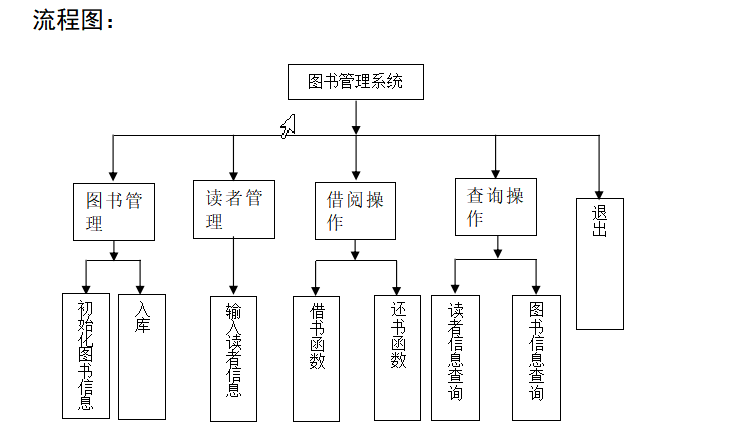接下来上源代码：
#include<bits/stdc++.h>
#define Max 3
#define k   2
typedef struct book{
char book_num;
char book_name;
char book_writer;
int book_kc;
int book_xy;
struct book *next;
}BK;
typedef struct borrow{
char borrow_book_num;
char limit_date;
}BO;
int right;
BO borrow[Max];
}RD;
BK *h_book;

void Init_book(){			//将图书信息进行初始化
BK *p0;
printf("\t\t\n欢迎使用本图书管理系统\n");
printf("\n请先初始化图书信息\n");
p0=(BK*)malloc(sizeof(BK));
h_book=p0;
printf("\n请输入图书信息:\n");
printf("书号:");
scanf("%s",&p0->book_num);
printf("书名:");
scanf("%s",&p0->book_name);
printf("著作者:");
scanf("%s",&p0->book_writer);
printf("库存量：");
scanf("%d",&p0->book_kc);
printf("现有量：");
scanf("%d",&p0->book_xy);
//  p0->book_xy=p0->book_kc;
p0->next=NULL;
printf("\n录入图书信息完成，按任意键继续下一步操作\n");
system("pause");
system("cls");
}

BK *p,*p0,*p1;
p=p1=h_book;
printf("\n新书入库模块\n");
printf("\n请输入新书信息\n");
p0=(BK *)malloc(sizeof(BK));
printf("书的编号:");
scanf("%s",&p0->book_num);
while(strcmp(p0->book_num,p1->book_num)!=0&&p1->next!=NULL)
p1=p1->next;
if(strcmp(p0->book_num,p1->book_num)==0){
printf("\n此编号图书已存在，直接入库。\n");
printf("新书库存量:");
scanf("%d",&p0->book_kc);
printf("新书现有量：");
scanf("%d",&p0->book_xy);
p1->book_kc+=p0->book_kc;
p1->book_xy+=p0->book_xy;
}
else{
printf("书名:");
scanf("%s",p0->book_name);
printf("书作者:");
scanf("%s",p0->book_writer);
printf("新书库存量:");
scanf("%d",&p0->book_kc);
printf("新书现有量：");
scanf("%d",&p0->book_xy);
while(p->next){
p=p->next;
}
if(h_book==NULL) h_book=p0;
else p->next=p0;
p0->next=NULL;
//      p0->book_xy=p0->book_kc;
}
printf("\n新书入库完成，按任意键继续下一步操作。\n");
system("pause");
system("cls");
}

RD *p,*p0 ;
p0=(RD *)malloc(sizeof(RD));
printf("\n请输入读者的信息:\n");
printf("读者书证号:");
printf("读者姓名:");
p0->right=0;
for(int i=0;i<Max;i++)
{
strcpy(p0->borrow[i].borrow_book_num,"0");
strcpy(p0->borrow[i].limit_date,"0");
}
//	p0->next=NULL;
else
{
while(p->next)
{
p=p->next;
}
p->next=p0;
}
p0->next=NULL;
//      p0->book_xy=p0->book_kc;
printf("\n添加读者完成，按任意键继续下一步操作。\n");
system("pause");
system("cls");
}

RD *p;
int i;
printf("\n读者信息查询模块\n");
printf("\n请输入你要查询读者编号:");
p=p->next;
printf("读者编号\t读者姓名"); 						//一个读者能借Max本书
printf("\n");
for(i=0;i<Max;i++){
printf("\n");
printf("书%d的编号",i+1);
printf("\t书%d的归还日期",i+1);
printf("\n");
printf("\t%s",p->borrow[i].borrow_book_num);
printf("\t\t%s",p->borrow[i].limit_date);
}
printf("\n");
}
else
printf("\n此读者编号不存在,请核对!按任意键返回...");
system("pause");
system("cls");
}

void Find_Book(){//查找图书信息
BK *p;
char find_book;
p=h_book;
printf("\n图书信息查询模块\n");
printf("\n请输入你要查询图书编号:");
scanf("%s",find_book);
while(strcmp(p->book_num,find_book)!=0&&p->next)
p=p->next;
if(strcmp(p->book_num,find_book)==0){
printf("\n图书编号:%s的信息为:\n",find_book);
printf("\n书编号\t\t书名\t作者\t现有量 \t库存量\n");
printf("%s\t\t%s\t%s\t%d\t%d\n",p->book_num,p->book_name,p->book_writer,p->book_xy,p->book_kc);
}
else
printf("\n此图书不存在,按任意键返回");
system("pause");
system("cls");
}

void Borrow_Book(){				//借书模块
BK *p0; RD *p1;
char bo_num,rea_num,lim_date;
int i;
printf("\n借书模块\n");
printf("\n请输入借书的读者编号:");
scanf("%s",rea_num);
p1=p1->next;
printf("\n此读者编号不存在!按任意键返回\n");
//system("pause");
//system("cls");
//return 0;
goto END;                                               //goto语句通常与条件语句配合使用,可用来实现条件转移,构成循环，跳出循环体等功能,这里是不存在跳出循环
}
printf("\n请输入你要借的书的编号:");
scanf("%s",bo_num);
while(strcmp(bo_num,p0->book_num)!=0&&p0->next!=NULL)       //遍历一次图书链表,判断此图书是否存在
p0=p0->next;
if(p0->next==NULL&&strcmp(bo_num,p0->book_num)!=0){         //如果存在,则继续,不存在就、则提示错误!
printf("\n此图书编号不存在!按任意键返回..\n");
goto END;
}
else if(p0->book_xy<=0){
printf("\n抱歉,此书已借完!请等待新书的到来!!\n按任意键返回....");
goto END;
}
else if(p1->right>=Max){
printf("\n不好意思,借书数目已足够多!不能再借书了!\n按任意键返回....");
goto END;
}
else if(strcmp(p1->borrow.borrow_book_num,"0")!=0){//此读者若已经借书,再判断此读者是否要借两本一样的书
for(i=0;i<Max;i++){        	            //遍历一次读者所借的书,如果发现有重复,则结束
if(strcmp(p1->borrow[i].borrow_book_num,bo_num)==0){
printf("\n抱歉!同一个读者不能同借两本相同的书!\n");
goto END;
}
}
}
for(i=0;i<Max;i++){
//测试：	printf("*****");
if(strcmp(p1->borrow[i].borrow_book_num,"0")==0){     //如果没有重复
printf("\n请输入你要归还图书的日期:");
scanf("%s",lim_date);
strcpy(p1->borrow[i].borrow_book_num,bo_num);     //记录所借书号,并且借书数目加一,现存量减一
strcpy(p1->borrow[i].limit_date,lim_date);
p1->right++;
p0->book_xy--;
goto END;
}
}
END:	system("pause");
system("cls");
}

void Return_Book(){//还书模块
BK *p; RD *q;
int i,j,find=0;
printf("\n还书模块\n");
printf("\n请输入要还书的读者编号:");
q=q->next;
find=2;                     //设置标志提前出错,则find=2,若借了,则find=1,若没借为find=0
printf("\n此读者编号不存在!按任意键返回..\n");
goto END;
}
printf("\n请输入读者还书的编号:");
scanf("%s",return_book_num);
while(p->next!=NULL&&strcmp(return_book_num,p->book_num)!=0)
p=p->next;
if(p->next==NULL&&strcmp(return_book_num,p->book_num)!=0){
find=2;                   //设置标志若提前出错,则find=2,若借了,则find=1,若没借为find=0
printf("\n错误!此图书编号不存在!按任意键返回..\n");
goto END;
}
for(i=0;i<Max;i++)
if(strcmp(return_book_num,q->borrow[i].borrow_book_num)==0){/*如果此读者借了此书*/
find=1;                  //设置标志若提前出错,则find=2,若借了,则find=1,若没借为find=0
for(j=i;j<Max-1;j++){
strcpy(q->borrow[j].borrow_book_num,q->borrow[j+1].borrow_book_num);
strcpy(q->borrow[j].limit_date,q->borrow[j+1].limit_date);
}
strcpy(q->borrow[j+1].borrow_book_num,"0");
strcpy(q->borrow[j+1].limit_date,"0");
p->book_xy++;
q->right--;
goto END;
}
if(find==0){
printf("\n错误!此读者未借此书!按任意键返回..\n");
goto END;
}
END: 	system("pause");
system("cls");
}

RD *p,*p0;
printf("\n文件保存失败!\n请重新启动本系统...\n");
exit(0);
}
while(p!=NULL){
printf("\n写入文件失败!\n请重新启动本系统!\n");
p0=p;
p=p->next;
free(p0);
}
}

void Save_Book(){ 				//保存图书信息到文件
FILE *fp_book;
BK *p,*p0;
p=h_book;
if((fp_book=fopen("Book.dat","wb"))==NULL){
printf("\n文件保存失败!\n请重新启动本系统...\n");
exit(0);
}
while(p!=NULL){
if(fwrite(p,sizeof(BK),1,fp_book)!=1)
printf("\n写入文件失败!\n请重新启动本系统!\n");
p0=p;
p=p->next;
free(p0);
}
h_book=NULL;
fclose(fp_book);
}

void Save(){ 					//保存读者和图书信息到文件
Save_Book();
}

{
RD *p1,*p2,*p3;
FILE *fp;
p1=(RD *)malloc(sizeof(RD));
while(! feof(fp)){
p1=(RD *)malloc(sizeof(RD));
p2->next=p1;
p3=p2;
p2=p1;
}
p3->next=NULL;
free(p1);
fclose(fp);
}

BK *p1,*p2,*p3;
FILE *fp;
fp=fopen("Book.dat","rb");
p1=(BK *)malloc(sizeof(BK));
h_book=p3=p2=p1;
while(! feof(fp)){
p1=(BK *)malloc(sizeof(BK));
p2->next=p1;
p3=p2;
p2=p1;
}
p3->next=NULL;
free(p1);
fclose(fp);
}

}

int sn;
printf("\n\t\t图书管理系统主菜单\n");
printf("*************************************************\n");
printf("*\t\t1----新书入库 \t\t\t*\n");
printf("*\t\t2----添加读者 \t\t\t*\n");
printf("*\t\t3----查询图书 \t\t\t*\n");
printf("*\t\t4----查询读者 \t\t\t*\n");
printf("*\t\t5----借书模块 \t\t\t*\n");
printf("*\t\t6----还书模块 \t\t\t*\n");
printf("*\t\t0----退出程序 \t\t\t*\n");
printf("*************************************************\n");
printf("请选择相应的代号:");
for(;;){
scanf("%d",&sn);
if(sn<0||sn>6)
printf("\n错误!请重新输入:");
else break;
}
return sn;
}

for(;;){
case 0:
system("cls");
Save();
printf("\n\n\t 数据保存成功！\n");
printf("\n\n\t欢迎下次使用本系统！\n");
printf("\n\n\t		By：XXX\n");
getchar();
exit(0);
case 3:Find_Book();  break;
case 5:Borrow_Book();break;
case 6:Return_Book();break;
default:printf("\n错误!");
exit(0);
}
}
}
main(){
Init_book();
}
}


运行截图：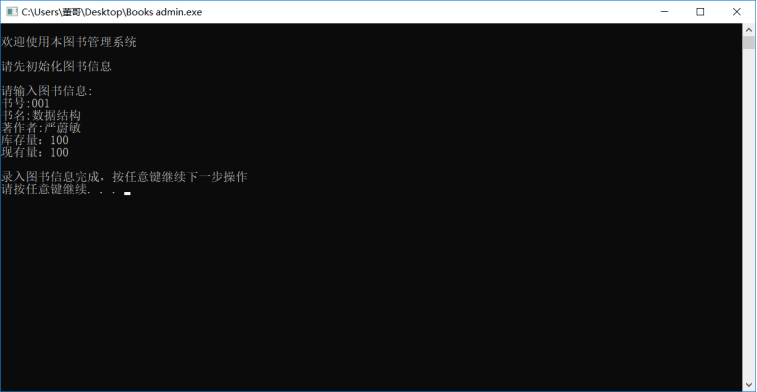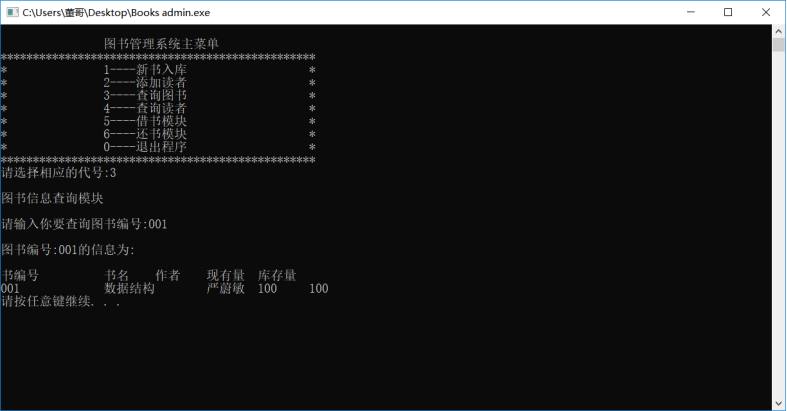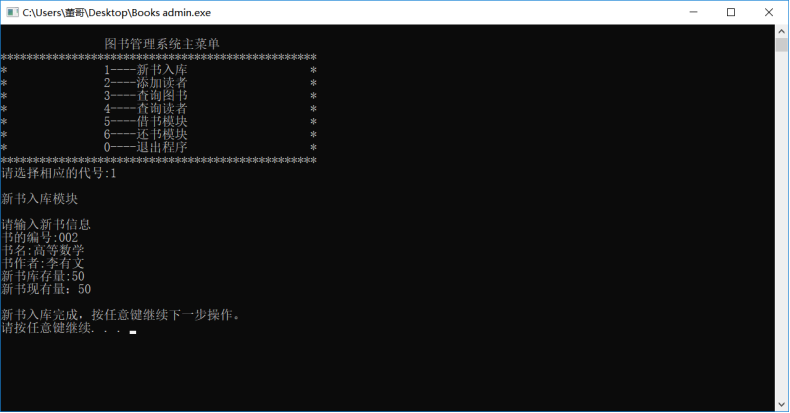课设说明书我后续会上传到附件里，当然仅供大家参考啊哈哈哈~ 大概是酱紫：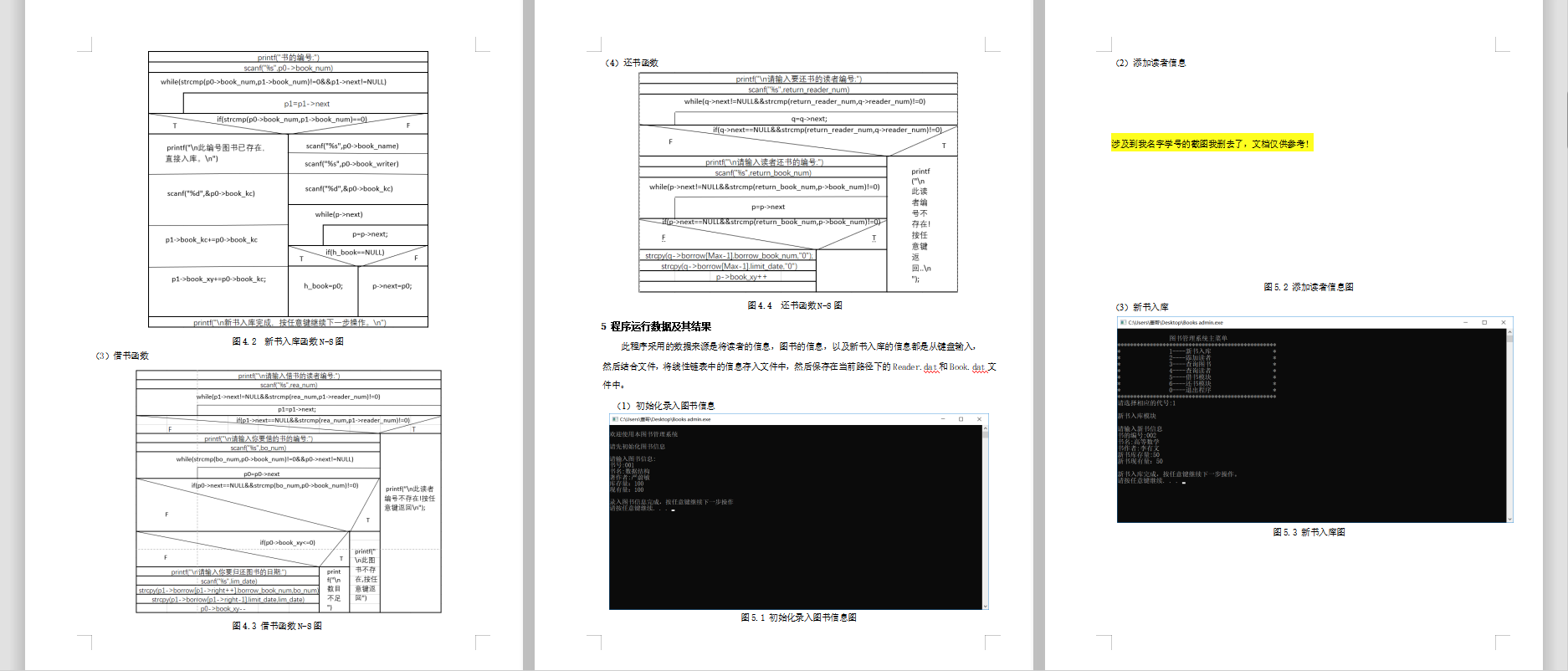Java课设的代码和文档等我开学了在写吧哈哈，最近忙着复习（预习？），因为疫情的原因，开学即考试！我太难了~ 大家有什么问题可以私信我~
展开全文• 数据结构课程设计，用C语言编写的一个小程序，内附源码和文档
• 2、删除员工模块：删除需要删除的员工的所有信息，通过自己定义的void del( )函数来实现。先通过查找找到需要删除的信息，将文件中除要删除的信息之外全部读入内存，删除原文件，将创建一个同名文件。3、修改模块：...
• 6/10/2020 仲恺农业工程学院 仲恺农业工程学院 仲 恺 农 业 工 程 学 院 仲 恺 农 业 工 程 学 院 课 程 设 计 报 告 课 程 设 计 报 告 题 目员工管理系统 题 目员工管理系统 数据结构与算法 课程名称 数据结构与...
• 数据结构做的员工管理系统，适合学生初学者，或是做课程设计的
•java源代码
• 在基于链表结构员工信息管理系统，有查找/录入/修改/删除/文件读写功能，小的的MFC初作，希望大家不吝赐教，更希望给大家带来帮助
• ## 员工信息管理系统

千次阅读 2018-04-23 10:04:36
在创建时由于手动找jar包态麻烦了，影响开发效率，所以我就用Maven 来管理项目结构。 主要的功能就是对员工信息进行添加，修改，分页查询和单个及批量删除 由于查询的时候带上了分页功能，这里就主要说一下查询吧...
• 利用数据结构的各种排序与查找算法实现的员工管理系统，数据存储在txt文件中。
• ## 班级考勤管理系统数据结构 C语言

千次阅读 热门讨论 2019-10-26 11:25:27
开发程序的工具Microsoft Visual C++ 6.0，（简称Visual C++ 6.0、VC++ 6.0或VC 6.0）是Microsoft公司推出的以C++语言为基础的开发Windows环境程序，面向对象的可视化集成编程系统。同时完全兼容C语言编程,它不但...
• 系统具有高效、易操作、易维护等特点，并且系统结构清晰、界面友好，功能较为齐全，能有效地协助管理人员进行人事管理工作。如果想真正把它用到实际的人事信息管理中，还需要读者对该系统进行一步的完善工作。access
• ## 学生宿舍信息管理系统

千次阅读 多人点赞 2020-12-31 15:20:16
本系统专门针对学校的公寓管理而开发的一个功能齐全、操作简单的学生公寓管理系统。适合学校的宿舍管理人员使用。本系统实现以下基本功能:管理员可以对公寓进行全程管理，不仅可以管理学生，还可以管理寝室人员及...
• ## 人员信息管理系统

千次阅读 2017-04-02 17:43:34
工厂模式，代理模式，MySQL数据库连接方式数据库连接方式：JDBC程序结构框架表的信息id int ；name varchar(50) ; sex varchar(10) ; birthday date基本类User类 定义user包含的信息。 定义get和set信息的方法。 Damysql...

# 数据结构员工信息管理系统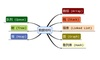数据结构 订阅